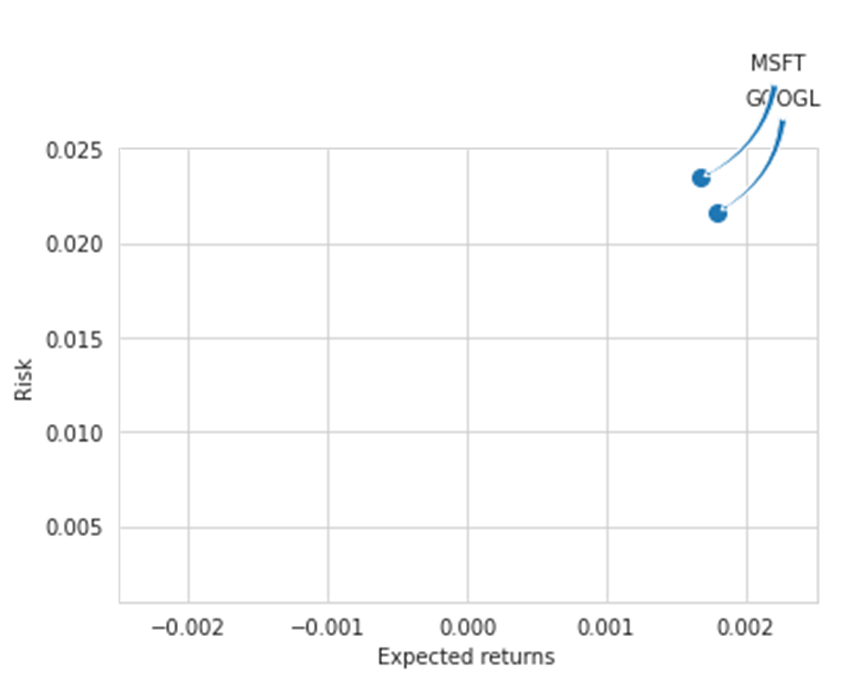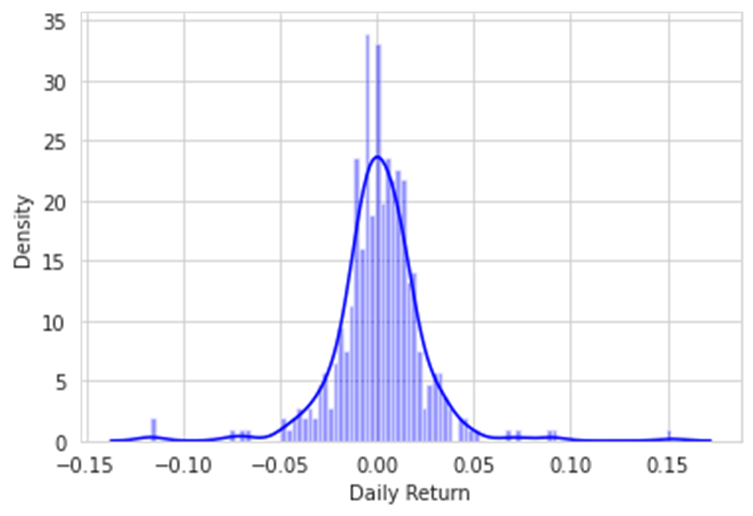top of page
Search

# Stock Market Analysis Using Yahoo Finance | Data Analysis Assignment Help

Introduction

Stock Market Analysis is one of the topic where data can be visualized to study different stocks given by Yahoo Finance. By getting an inference from the data, we can analyze the gigantic stocks through different visualization tools used in python and check out different aspects and risk assessment of a stock based on its previous performances. Here, we have studied three different stocks such as Apple, Google and Microsoft. We will analyze their values from Nov 2019 to May 2021 regarding basic attributes for the stock information, visualizing the opening and closing prices, volume traded on each day, daily return analysis, correlations with other stocks, risk stuffs, values that are at risk using the Monte Carlo Concept.

Perquisites

Libraries such as Numpy, Pandas, and DataReader are needed to be installed during the implementation and visualization for the stocks data. We will be using Yahoo Finance data to study Apple, Microsoft and Google stocks.

Stock Information Analysis

Analysis is divided into different sections which are as follows:

1. Basic Attributes of Stock Analysis

While analyzing the data for all the three stocks , we studied the volume and closing price of each stock through the plots in which the following figure shows the period of huge peaks of volume and closing and also give information about the Moving AverageAAPL ClosingGOOGL ClosingMSFT ClosingGOOGL VolumeMSFT VolumeMoving Averages

AAPL :GOOGL:MSFT:2. Daily Return Analysis

Following are the graphs that illustrate daily return values of all the stocks where y axis denotes the percentage change for every day and x axis denotes the period time.GOOGL:MSFT:After quick analysis done on actual numerical values through pairgrid and heatmap , we saw that for closing price there is a strong correlation between AAPL and MSFT and for the daily returns, there is a correlation between strong correlation between GOOGL and MSFT.

3. Risk Analysis

To quantify the risk occurred, we have used the information as daily percentage returns by comparing the expected return with standard deviation of daily returns.We can say these stocks (MSFT, GOOGL) have lower risk and positive expected returns.

4. Value at Risk

Determination of risk parameter for all the stocks mentioned here. We can treat value at risk as the amount of money we could expect to lose (aka putting at risk) for a given confidence interval.

Empirical Quantile of daily returns for stocks5. Value at Risk Using Monte Carlo Method

Using the Monte Carlo to run many trials with random market conditions, then we'll calculate portfolio losses for each trial. After this, we'll use the aggregation of all these simulations to establish how risky the stock is.

Following are the graphs to check all the stocks regarding it by using the stock monte carlo analysis which takes shocks and drift dataApart from this, we also performed simulations regarding 1% empirical quantile regarding each stockThis basically means for every initial GOOGL stock you purchase you're putting about \$55.81 at risk 99% of the time from our Monte Carlo SimulationThis basically means for every initial AAPL stock you purchase you're putting about \$2.8 at risk 99% of the time from our Monte Carlo Simulation.This basically means for every initial MSFT stock you purchase you're putting about \$6.69 at risk 99% of the time from our Monte Carlo Simulation.

Also we have estimated the Value at Risk for other domains such as

• Johnson & Johnson > JNJ (U.S.: NYSE) JNJ

• Wal-Mart Stores Inc. > WMT (U.S.: NYSE) WMT

• Nike Inc. > NKE (U.S.: NYSE) NKE

Following are the graphs to check the closing price of JNJ, NKE and WMT6. Value at Risk using Bootstrap Method

We will calculate the empirical quantiles from a histogram of daily returns. Following are the graphs for getting the empirical quantile understanding regarding other domains.The 0.05 empirical quantile of JNJ stock daily returns is at -0.02365349403576538. That means that with 95% confidence, our worst daily loss will not exceed 1%. If we have a 1 million dollar investment, our one-day 5% VaR is 0.023 * 1,000,000 = \$23,000.

MWT:The 0.05 empirical quantile of WMT stock daily returns is at -0.020. That means that with 95% confidence, our worst daily loss will not exceed 1.3%. If we have a 1 million dollar investment, our one-day 5% VaR is 0.020 * 1,000,000 = \$20,000.

NKE :The 0.05 empirical quantile of NKE stock daily returns is at -0.030. That means that with 95% confidence If we have a 1 million dollar investment, our one-day 5% VaR is 0.030 * 1,000,000 = \$30,000.

Conclusion:

The stocks that are studied here such as AAPL, GOOGL and MSFT have high technical analysis as they have high variations during the period from November 2019 to March 2021. Even they are strongly correlated as there work and technologies are dependent on each other and so they show a positive relationship and also with respect to daily returns and risks calculated, MSFT and GOOGL plays a major role in giving more returns at lower risks. Further analysis can also be done to know more future patterns through monte carlo method.